#### Chapter 1 Real Numbers R.D. Sharma Solutions for Class 10th Math Exercise 1.5

Exercise 1.5

Level 1

1. Show that the following numbers are irrational.
(i) 1/√2
(ii) 7√5
(iii) 6+√2
(iv) 3-√5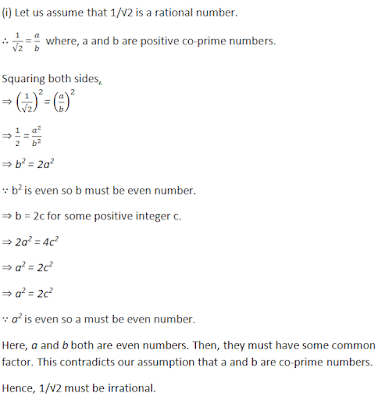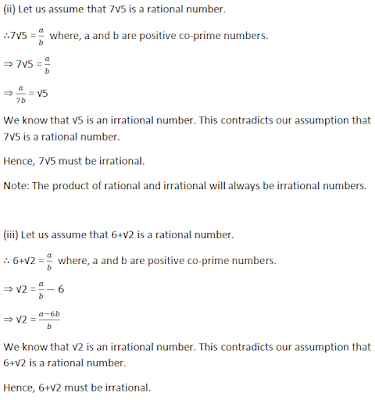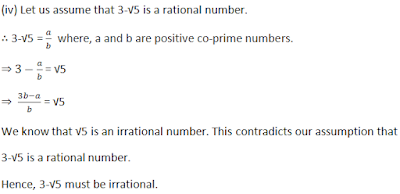2. Prove that following numbers are irrationals.
(i) 2/√7
(ii) 3/2√5
(iii) 4+√2
(iv) 5√2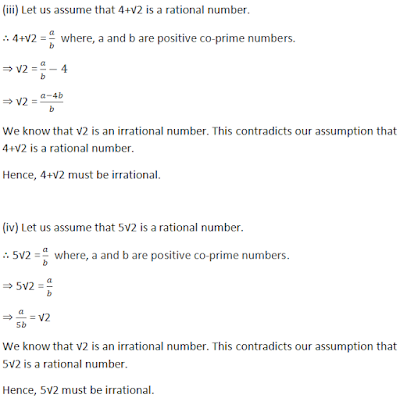3. Show that 2-√3 is an irrational number.4. Show that 3+√2 is an irrational number.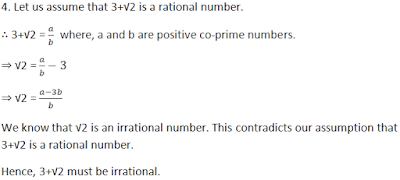5. Prove that 4-√5 is an irrational number.6. Show that 5-2√3 is an irrational number.7. Prove that 2√3-1 is an irrational number.8. Prove that 2-3√5 is an irrational number.9. Prove that √5+√3 is irrational.10. Prove that √2+√3 is an irrational number.Level 2

11. Prove that for any prime positive integer p, √p is an irrational number.